Foxtable(狐表)用户栏目专家坐堂 → EXCEL的问题

共有96人关注过本帖树形打印复制链接

# 主题：EXCEL的问题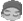hongye
1楼 | 信息 | 搜索 | 邮箱 | 主页 | UC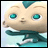加好友发短信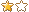EXCEL的问题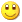Post By：2021/4/8 15:36:00 [只看该作者]

 Dim FirstRow As Integer = Ws.Range("A1").End(MSExcel.XlDirection.xlDown).Row'对A列从第1行开始向下查找,直到找到最后一个非空单元格为止,并得到其行号.也就是有内容的开始行这个是找到最后一个非空行，那如何找到最后一个非空列呢？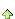2楼 | 信息 | 搜索 | 邮箱 | 主页 | UC加好友发短信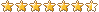Post By：2021/4/8 15:38:00 [只看该作者]hongye
3楼 | 信息 | 搜索 | 邮箱 | 主页 | UC加好友发短信Post By：2021/4/8 15:59:00 [只看该作者]

 Dim dlg As New OpenFileDialog '定义一个新的OpenFileDialogdlg.Filter= "Excel文件|*.xlsx" '设置筛选器If dlg.ShowDialog = DialogResult.Ok Then '如果用户单击了确定按钮    MessageBox.Show("你选择的是:" & dlg.FileName,"提示") '提示用户选择的文件Dim App As New MSExcel.ApplicationDim Wb As MSExcel.WorkBook = App.WorkBooks.Open(dlg.FileName)Dim Ws As MSExcel.WorkSheet = Wb.WorkSheets(1)Dim Rg As MSExcel.Range = Ws.UsedRangeDim FirstRow As Integer = Ws.Range("A1").End(MSExcel.XlDirection.xlDown).RowDim FirstCol As Integer = ws.Range("iv1").End(MsExcel.XlDirection.xlToRight).Columnmsgbox(FirstRow & "," & FirstCol)End If结果 列为16384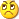4楼 | 信息 | 搜索 | 邮箱 | 主页 | UC加好友发短信Post By：2021/4/8 16:06:00 [只看该作者]

 Dim Ws As MSExcel.WorkSheet = Wb.WorkSheets(1)Dim rowsmax As Integer = 0Dim colmax As Integer = 0Dim rg = Ws.UsedRange'Dim FirstRow As Integer = Ws.Range("A1:d1").End(MSExcel.XlDirection.xlDown).Row'msgbox(firstrow)For i As Integer =1 To rg.Columns.count        Dim r = ws.cells(1000,i).End(MsExcel.XlDirection.xlUp).Row    If r > RowsMax Then        RowsMax = r    End IfNextFor i As Integer = 1 To rowsMax        Dim r = ws.cells(i,200).End(MsExcel.XLDirection.xlToLeft).Column    If r > ColMax  Then        ColMax = r    End IfNextmsgbox(RowsMax & "," & ColMax)hongye
5楼 | 信息 | 搜索 | 邮箱 | 主页 | UC加好友发短信Post By：2021/4/9 14:48:00 [只看该作者]

 Dim dlg As New OpenFileDialog '定义一个新的OpenFileDialogdlg.Filter= "Excel文件|*.xlsx" '设置筛选器If dlg.ShowDialog = DialogResult.Ok Then '如果用户单击了确定按钮    MessageBox.Show("你选择的是:" & dlg.FileName,"提示") '提示用户选择的文件    Dim Str1 As String = dlg.FileName    Dim fx As String    If Str1 > "" AndAlso Str1.Contains("返修") Then        fx = "返修单"    End If    Dim khmc As String    khmc = FileSys.GetName(dlg.FileName).SubString(0,3)    msgbox(khmc)    Dim App As New MSExcel.Application    Dim Wb As MSExcel.WorkBook = App.WorkBooks.Open(dlg.FileName)    Dim Ws As MSExcel.WorkSheet = Wb.WorkSheets(1)    Dim Rg1 As MSExcel.Range    Dim rowsmax As Integer = 0    Dim colmax As Integer = 0    Dim x As String    Dim y As String    Dim x1 As String    Dim y1 As String    Dim x2 As String    Dim y2 As String    Dim chrq As String    Dim rg = Ws.UsedRange    For i As Integer =1 To rg.Columns.count        Dim r = ws.cells(1000,i).End(MsExcel.XlDirection.xlUp).Row        If r > RowsMax Then            RowsMax = r        End If    Next    For i As Integer = 1 To rowsMax        Dim r = ws.cells(i,200).End(MsExcel.XLDirection.xlToLeft).Column        If r > ColMax  Then            ColMax = r        End If    Next    For i As Integer = 1 To RowsMax        For j As Integer = 1 To ColMax            Rg1 = Ws.Cells(i,j)            If Rg1.text = "箱号" Then                x = i                y = j            End If        Next    Next    For i As Integer = 1 To RowsMax        For j As Integer = 1 To ColMax            Rg1 = Ws.Cells(i,j)            If Rg1.text = "总计:" Then                x1 = i                y1 = j            End If        Next    Next    For i As Integer = 1 To RowsMax        For j As Integer = 1 To ColMax            Rg1 = Ws.Cells(i,j)            If Rg1.text.Contains("出货日期") Then                x2 = i                y2 = j                Dim chr As String                chr = Ws.Cells(i,j).Value.SubString(5).Trim(" ").Replace("/","-")                Dim Day As Date = chr                chrq = Format(Day,"d")                msgbox(chrq)            End If        Next    Next    msgbox("箱号位置在" & x & "," & y & "总计位置在" & x1 & "," & y1 & "出货日期位置在" & x2 & "," & y2 )    Dim Builder As New ADOXBuilder    Dim tbl As ADOXTable    Builder.Open()    tbl = Builder.NewTable("临时箱单") '创建表    With tbl        For j As Integer = y To ColMax            Rg1 = Ws.Cells(x,j)            Dim bt As String = Rg1.text.Trim(" ").Replace("/","")            .AddColumn(bt,ADOXType.String, 25)            msgbox(bt)        Next    End With    Builder.AddTable(tbl) '增加表    Builder.Close()    End If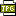此主题相关图片如下：01.jpg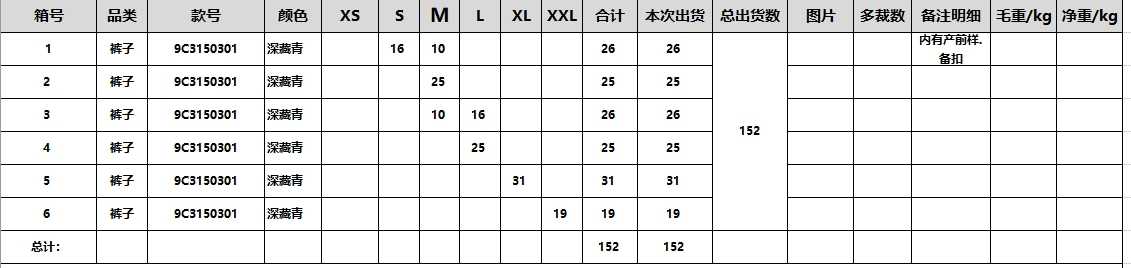新增表已完成，1、请问怎么把EXCEL里的数据填入对应的数据列里呢？如何编写这个代码？2、这个代码运行好像比较慢这是为什么？6楼 | 信息 | 搜索 | 邮箱 | 主页 | UC加好友发短信Post By：2021/4/9 14:59:00 [只看该作者]

 1、参考：http://www.foxtable.com/bbs/dispbbs.asp?boardid=2&Id=1356842、vba一向都不快，何况还遍历了N次表格的所有单元格hongye
7楼 | 信息 | 搜索 | 邮箱 | 主页 | UC加好友发短信Post By：2021/4/9 15:10:00 [只看该作者]

 你提供的参考我看了，但是有一定的区别，主要是我的数据表列是按照EXCEL生成的，在生成时不知道列名，请问怎么做？8楼 | 信息 | 搜索 | 邮箱 | 主页 | UC加好友发短信Post By：2021/4/9 15:20:00 [只看该作者]

 遍历第一行所有列获取标题看：http://www.foxtable.com/bbs/dispbbs.asp?BoardID=2&ID=105144&replyID=&skin=1http://www.foxtable.com/bbs/dispbbs.asp?boardid=2&Id=95427hongye
9楼 | 信息 | 搜索 | 邮箱 | 主页 | UC加好友发短信Post By：2021/4/9 16:23:00 [只看该作者]

 Dim dlg As New OpenFileDialog '定义一个新的OpenFileDialogdlg.Filter= "Excel文件|*.xlsx" '设置筛选器If dlg.ShowDialog = DialogResult.Ok Then '如果用户单击了确定按钮    MessageBox.Show("你选择的是:" & dlg.FileName,"提示") '提示用户选择的文件    Dim Str1 As String = dlg.FileName    Dim fx As String    If Str1 > "" AndAlso Str1.Contains("返修") Then        fx = "返修单"    End If    Dim khmc As String    khmc = FileSys.GetName(dlg.FileName).SubString(0,3)    msgbox(khmc)    Dim App As New MSExcel.Application    Dim Wb As MSExcel.WorkBook = App.WorkBooks.Open(dlg.FileName)    Dim Ws As MSExcel.WorkSheet = Wb.WorkSheets(1)    Dim Rg1 As MSExcel.Range    Dim rowsmax As Integer = 0    Dim colmax As Integer = 0    Dim x As String    Dim y As String    Dim x0 As String    Dim y0 As String    Dim x1 As String    Dim y1 As String    Dim x2 As String    Dim y2 As String    Dim chrq As String    Dim rg = Ws.UsedRange    For i As Integer =1 To rg.Columns.count        Dim r = ws.cells(1000,i).End(MsExcel.XlDirection.xlUp).Row        If r > RowsMax Then            RowsMax = r        End If    Next    For i As Integer = 1 To rowsMax        Dim r = ws.cells(i,200).End(MsExcel.XLDirection.xlToLeft).Column        If r > ColMax  Then            ColMax = r        End If    Next    For i As Integer = 1 To RowsMax        For j As Integer = 1 To ColMax            Rg1 = Ws.Cells(i,j)            If Rg1.text = "箱号" Then                x = i                y = j            End If        Next    Next    For i As Integer = 1 To RowsMax        For j As Integer = 1 To ColMax            Rg1 = Ws.Cells(i,j)            If Rg1.text = "本次出货" Then                x0 = i                y0 = j            End If        Next    Next    For i As Integer = 1 To RowsMax        For j As Integer = 1 To ColMax            Rg1 = Ws.Cells(i,j)            If Rg1.text = "总计:" Then                x1 = i                y1 = j            End If        Next    Next    For i As Integer = 1 To RowsMax        For j As Integer = 1 To ColMax            Rg1 = Ws.Cells(i,j)            If Rg1.text.Contains("出货日期") Then                x2 = i                y2 = j                Dim chr As String                chr = Ws.Cells(i,j).Value.SubString(5).Trim(" ").Replace("/","-")                Dim Day As Date = chr                chrq = Format(Day,"d")                msgbox(chrq)            End If        Next    Next    msgbox("箱号位置在" & x & "," & y & "总计位置在" & x1 & "," & y1 & "出货日期位置在" & x2 & "," & y2 )    Dim Builder As New ADOXBuilder    Dim tbl As ADOXTable    Builder.Open()    tbl = Builder.NewTable("临时箱单") '创建表    With tbl        For j As Integer = y To y0            Rg1 = Ws.Cells(x,j)            Dim bt As String = Rg1.text.Trim(" ").Replace("/","")            .AddColumn(bt,ADOXType.String, 25)            msgbox(bt)        Next    End With    Builder.AddTable(tbl) '增加表    Builder.Close()    Dim Book As New XLS.Book("I:\transDetail2021-03-24.xls")    Dim Sheet As XLS.Sheet = Book.Sheets(0)    Tables("临时箱单").StopRedraw()    For Each dc As DataCol In DataTables("临时箱单").DataCols        Dim nms As String = dc.Name        For n As Integer = x+1 To x1            Dim r As Row = Tables("临时箱单").AddNew()            For m As Integer = y To y0                r(nms(m)) = Sheet(n,m).Value            Next        Next        Tables("临时箱单").ResumeRedraw()    NextEnd If好像这句话错了，你帮我看看10楼 | 信息 | 搜索 | 邮箱 | 主页 | UC加好友发短信Post By：2021/4/9 16:35:00 [只看该作者]

 提示什么错误？总数 17 1 2 下一页